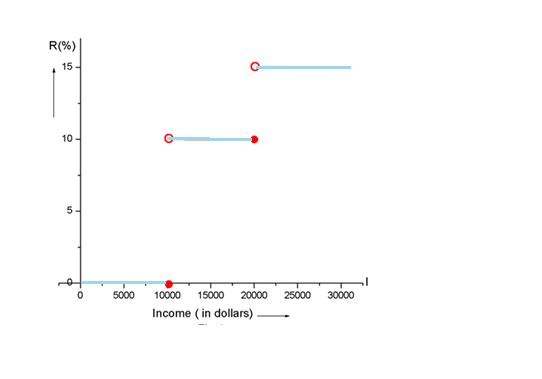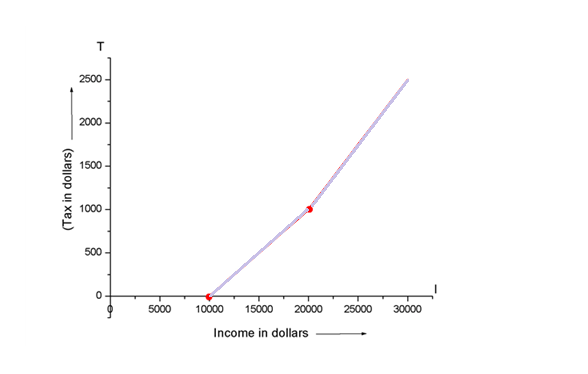# Sketch the graph of the tax rate R as a function of the income I .### Single Variable Calculus: Concepts...

4th Edition
James Stewart
Publisher: Cengage Learning
ISBN: 9781337687805### Single Variable Calculus: Concepts...

4th Edition
James Stewart
Publisher: Cengage Learning
ISBN: 9781337687805

#### Solutions

Chapter 1.1, Problem 61E

a.

To determine

## Sketch the graph of the tax rate R as a function of the income I .

Expert Solution### Explanation of Solution

Given information:

In a certain country, income tax is assessed as follows. There is no tax on income up to $10,000 . Any income over$10,000 is taxed at rate of 10% , up to an income of $20,000 . Any income over$20,000 is taxed at 15% .

Sketch the graph of the tax rate R as a function of the income I .

Calculation:

Suppose R be the rate of tax and I be the income, so R is the function of I .

Now we have been given that, there is no tax income up to $10,000 So R(I)=0 for I$10,000

If the income I is in the interval $10000<I$20000 , then the tax rate is 10%

So R(I)=10 (%) for $10000<I$20000

If the income is more than $20000 then the tax rate is 15% Then R(I)=15 (%) for I>$20000

Now we can write the tax rate R as a function of I as follows

R(I)={0(%),for,I$1000010(%),for,$10000<I$2000015(%),for,I>$20000

Now we can draw a graph,b.

To determine

### How much tax is assessed on an income of $14,000 on$26,000 ?

Expert Solution

$14000=$400 and  $26000=$1900

### Explanation of Solution

Given information:

In a certain country, income tax is assessed as follows. There is no tax on income up to $10,000 . Any income over$10,000 is taxed at rate of 10% , up to an income of $20,000 . Any income over$20,000 is taxed at 15% .

How much tax is assessed on an income of $14,000 on$26,000 ?

Calculation:

We have to calculate tax on income of $14,000 There is no tax if income I$10000

So non-taxable income is = $10000 Then taxable income is = 14000010000 dollars=$4000

Since the total income is in the interval (10000,20000)

So the income tax = 10% of 4000

=4000×10100=$400 Hence, tax on income of$14000=$400 Now, we have to calculate the tax on income of$26,000

First we have to break this

$26000=$10000+$10000+$6000

Tax on first $10000=0 Tax on second$10000=10%of$10000 Tax on last$6000=15%of$6000 Then total tax on income of$26000

=10000×0+10000×0.1+6000×0.15 dollars

=$1900 Hence, the tax on income of$26000=$1900 c. To determine ### Sketch the graph of the total assessed tax T as a function of the income I . Expert Solution ## Answer to Problem 61E### Explanation of Solution Given information: In a certain country, income tax is assessed as follows. There is no tax on income up to$10,000 . Any income over $10,000 is taxed at rate of 10% , up to an income of$20,000 . Any income over $20,000 is taxed at 15% . Sketch the graph of the total assessed tax T as a function of the income I . Calculation: Since there is no tax if income I$10000 , so graph of tax T(I) will start from the point (10000,0)

If the income I>$10000 and I$20000 , then tax rate is 10%

Maximum taxable income in the interval is $20000$10000=$10000 Then maximum tax in this interval = 10000×0.1=$1000

Hence curve passes through the point (20000,1000)

Now if income tax I>\$20000 , then the tax rate is 15%

So tax T (I) = (120000)×0.15+1000 dollars

Or T(I)=0.15I2000 dollars

Hence the graph of tax T(I) is as follows### Have a homework question?

Subscribe to bartleby learn! Ask subject matter experts 30 homework questions each month. Plus, you’ll have access to millions of step-by-step textbook answers!